# Container with water

The cube-shaped container is filled with water to half its height. If we add 20 liters of water, the container will be filled to three-quarters of its height. What is the volume of the whole container?

V =  80 l

### Step-by-step explanation: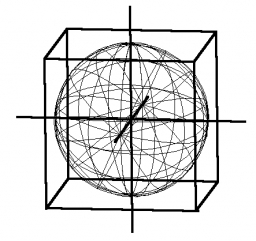Did you find an error or inaccuracy? Feel free to write us. Thank you!Tips to related online calculators
Need help to calculate sum, simplify or multiply fractions? Try our fraction calculator.
Do you have a linear equation or system of equations and looking for its solution? Or do you have a quadratic equation?
Tip: Our volume units converter will help you with the conversion of volume units.

## Related math problems and questions:

• Water containerThe cube-shaped container is filled to two-thirds of its height. If we pour 18 liters, it will be filled to three-fifths of the height. What is the volume of the whole container?
• Cube-shaped containerThe cube-shaped container has a height of 52 cm and a square base. The container was filled to the brim with water, then we immersed a metal cube in it, which caused 2.7 l of water to flow out of the container. After removing the cube from the water, the
• Water overflow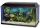A rectangular container that has a length of 30 cm, a width of 20 cm, and a height of 24 cm is filled with water to a depth of 15 cm. When an additional 6.5 liters of water are poured into the container, some water overflows. How many liters of water over
• Cylinder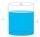The cylinder-shaped container has 80 liters of water. The water reaches 45 cm height. How many water liters will be in a container if the water level extends to a height of 72 cm? Write the result in liters, write down only as a whole or decimal number.
• Half-filled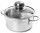A cylindrical pot with a diameter of 24 cm is half-filled with water. How many centimeters will the level rise if we add a liter of water to it?
• Barrel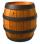The barrel is filled with water up to 57% of its capacity. When we add two buckets will be filled to 73% of its capacity. What is the volume of a barrel, if the volume of the bucket is 12 liters?
• Mineral water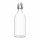The bottle contains 1.5 liters of mineral water. Pour all the water from the bottle into empty cups with a volume of 1/3 l. All but one will be filled to the brim. What part of the volume of the last cup is filled with water?
• Cylinder container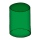If the cylinder-shaped container is filled with water to a height of 5 dm, it contains 62.8 hectoliters of water. Calculate the diameter of the bottom of the container. Use the value π = 3.14.
• Wooden containerThe locksmith should cover the cube-shaped wooden container with a metal sheet inside. The outer edge of the container is 54cm. The wall thickness is 25 mm. The container has no lid. Calculate. How many sheets will be needed to cover it?
• Three cubesTwo cube-shaped boxes with edges a = 70 cm; b = 90 cm must be replaced by one cube-shaped box. What will be its edge?
• Cylinder-shaped vase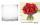If the cylinder-shaped vase is filled with water up to 35 cm, it contains 1 liter of water. How much water will it contain if it is filled to a height of 45cm?
• Water levelThe glass container has a cuboid shape with dimensions of the bottom 24 cm and 12 cm. The height of the water is 26 cm. Calculate the body's volume, which is sunk into the water if the water level rise by 3 cm.
• ContainerThe container-shaped box with internal dimensions of 3.9 m, 3.25 m and 2.6 m was completely filled with goods in the same cubic boxes. How long edge could this box have?
• Three-quarters of its volume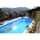The pool has a block shape with a length of 8m, a width of 5.3m and a depth of 1.5m. How many hl of water is in it if it is filled to three-quarters of its volume?
• Cube-shaped boxThe cube-shaped box is filled to the brim with 2 liters of milk. Calculate the edge and surface of the box.
• Water level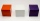How many cm will the water level in a cube-shaped tank with an edge of 3 m drop if we discharge 189 hl of water?
• Mr. Gardener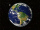Mr. Gardener wants to make wood for the balcony. Boxes. Each will have the shape of a perpendicular prism with a square base, the height is limited to 60 cm. Each container will be filled with soil by pouring the whole bag of substrate sold in a package w